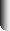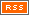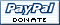``` The Sound of Friction (to the tune of 'Sound of Silence') by Ken Ferrier and Antoni Chan ``` ``` Hello friction my old friend I've come to ponder you again Because this problem that I am doing Requires an object to be moving And this problem Has caused me to beat my brain Against the wall It makes the sound of friction You see I've got this blocky thing Resting on a coffee table The tables's tilted at 0 degrees So I can calculate cosine with ease And the block has a mass of 20 kilograms It's made of spam Which is short for spiced ham So I've got this block of spam And it's attached to a rope And the rope goes over a pulley At the edge of the coffee table And the other side of the rope is attached to a fish The fish is dead It has a mass of X The question tells us to find X So that the block slides off the table And it tells us that spam has a Mu Of point five when it is at rest And you ask, "Hey, what the Hell is Mu?" It occurs to you That you know nothing... about fiction Well, friction is a force Opposing the motion It depends on the mass of the block And not on the surface area And also on the coefficient of friction Which is just a property Of the two... Materials The equation goes like this: Friction equals N times Mu Where N is the normal force Acting orthogonally to the plane And N is subject to Newton's Second Law F equals M A And here A is... Acceleration due to gravity So now we calculate the force Needed to exceed friction Recall that friction equals N times Mu And N equals M times A And then, you multiply by the constant Mu Which is point five And your answer... Is 98 Newtons So the fish must cause a force Greater than 98 Newtons And we calculate X this way 98 is less than X times A Where A is the acceleration due to gravity The answer says That X is greater... than 10 kilograms And now you know all about friction And how to apply it to the world If you ever have an inclined plane Underneath a block of Spam And the spam is connected to a fish You'll know what to do. You'll say Physics... I love you. ```Site MapHuge Huge! © 2005